# Extremal properties of polynomials

Jump to: navigation, search

Properties of algebraic, trigonometric or generalized polynomials that distinguish them as solutions of some extremal problems.

For example, the Chebyshev polynomials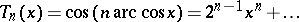have minimal norm in the spaceamong all algebraic polynomials of degreewith leading coefficient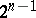(P.L. Chebychev, 1853); therefore they are the solution of the extremal problemIn other words, the polynomialdiffers least from zero in the spaceamong all polynomials of degreewith leading coefficient equal to.

Extremal problems in spaces of polynomials are mainly studied in the spaces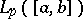,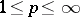. In this context most of the available results are connected with the cases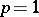, 2 and(the metric of). In particular, these metrics are used to find the explicit form of the polynomials that differ least from zero. In the metric of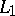these are the Chebychev polynomials of the second kind, in the metric ofone obtains the Legendre polynomials; concerning the metric ofsee above. The set of classical orthogonal polynomials deviating least from zero in a weighted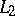-space (Laguerre polynomials; Hermite polynomials; Jacobi polynomials; etc.) has also been described.

E.I. Zolotarev (1877) considered the question of determining polynomials of the form(with two fixed leading coefficients) that deviate least from zero in the metric of. He found a one-parameter family of polynomials that solved this problem, and expressed them in terms of elliptic functions.

The Chebychev polynomials are extremal in the problem of an inequality for the derivatives; namely, the exact Markov inequality (where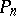is a polynomial of degree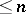)(*)

holds, with equality for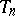. Inequality (*) was proved by A.A. Markov (1889) for, and by V.A. Markov (1892) for all other values of. Concerning a similar inequality for trigonometric polynomials see Bernstein inequality.

Some extremal properties of algebraic and trigonometric polynomials in the uniform metric carry over to Chebychev systems of functions (see ). Concerning the theory of extremal problems and extremal properties of polynomials see .